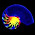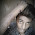## December 1, 2011

### The Great Mystery of Linear Gradient Lighting

A long, long time ago, in pretty much the same place I'm sitting in right now, I was learning how one would do 2D lighting with soft shadows and discovered the age old adage in 2D graphics: linear gradient lighting looks better than mathematically correct inverse square lighting.

Strange.

I brushed it off as artistic license and perceptual trickery, but over the years, as I dug into advanced lighting concepts, nothing could explain this. It was a mystery. Around the time I discovered microfacet theory I figured it could theoretically be an attempt to approximate non-lambertanian reflectance models, but even that wouldn't turn an exponential curve into a linear one.

This bizarre law even showed up in my 3D lighting experiments. Attempting to invoke the inverse square law would simply result in extremely bright and dark areas and would look absolutely terrible, and yet the only apparent fix I saw anywhere was simply calculating light via linear distance in clear violation of observed light behavior. Everywhere I looked, people calculated light on a linear basis, everywhere, on everything. Was it the equations? Perhaps the equations being used operated on linear light values instead of exponential ones and so only output the correct value if the light was linear? No, that wasn't it. I couldn't figure it out. Years and years and years would pass with this discrepancy left unaccounted for.

A few months ago I noted an article on gamma correction and assumed it was related to color correction or some other post process effect designed to compensate for monitor behavior, and put it as a very low priority research point on my mental to-do-list. No reason fixing up minor brightness problems until your graphics engine can actually render everything properly. Yesterday, though, I happened across a Hacker News posting about learning modern 3D engine programming. Curious if it had anything I didn't already know, I ran through its topics, and found this. Gamma correction wasn't just making the scene brighter to fit with the monitor, it was compensating for the fact that most images are actually already gamma-corrected.

In a nutshell, the brightness of a monitor is exponential, not linear (with a power of about 2.2). The result is that a linear gradient displayed on the monitor is not actually increasing in brightness linearly. Because it's mapped to a curve, it will actually increase in brightness exponentially. This is due to the human visual system processing luminosity on a logarithmic scale. The curve in question is this:Source: GPU Gems 3 - Chapter 24: The Importance of Being Linear

You can see the effect in this picture, taken from the article I mentioned:The thing is, I always assumed the top linear gradient was a linear gradient. Sure it looks a little dark, but hey, I suppose that might happen if you're increasing at 25% increments, right? WRONG. The bottom strip is a true linear gradient1. The top strip is a literal assignment of linear gradient RGB values, going from 0 to 62 to 126, etc. While this is, digitally speaking, a mathematical linear gradient, what happens when it gets displayed on the screen? It gets distorted by the CRT Gamma curve seen in the above graph, which makes the end value exponential. The bottom strip, on the other hand, is gamma corrected - it is NOT a mathematical linear gradient. It's values go from 0 to 134 to 185. As a result, when this exponential curve is displayed on your monitor, it's values are dragged down by the exact inverse exponential curve, resulting in a true linear curve. An image that has been "gamma-corrected" in this manner is said to exist in sRGB color space.

The thing is, most images aren't linear. They're actually in the sRGB color space, otherwise they'd look totally wrong when we viewed them on our monitors. Normally, this doesn't matter, which is why most 2D games simply ignore gamma completely. Because all a 2D engine does is take a pixel and display it on the screen without touching it, if you enable gamma correction you will actually over-correct the image and it will look terrible. This becomes a problem with image editing, because digital artists are drawing and coloring things on their monitors and they try to make sure that everything looks good on their monitor. So if an artist were visually trying to make a linear gradient, they would probably make something similar to the already gamma-corrected strip we saw earlier. Because virtually no image editors linearize images when saving (for good reason), the resulting image an artist creates is actually in sRGB color space, which is why only turning on gamma correction will usually simply make everything look bright and washed out, since you are normally using images that are already gamma-corrected. This is actually good thing due to subtle precision issues, but it creates a serious problem when you start trying to do lighting calculations.

The thing is, lighting calculations are linear operations. It's why you use Linear Algebra for most of your image processing needs. Because of this, when I tried to use the inverse-square law for my lighting functions, the resulting value that I was multiplying on to the already-gamma-corrected image was not gamma corrected! In order to do proper lighting, you would have to first linearize the gamma-corrected image, perform the lighting calculation on it, and then re-gamma-correct the end result.

Wait a minute, what did we say the gamma curve value was? It's $x^{2.2}$, so $x^{0.45}$ will gamma-correct the value $x$. But the inverse square law states that the intensity of a light is actually $\frac{1}{x^2}$, so if you were to gamma correct the inverse square law, you'd end up with: ${\bigg(\frac{1}{x^2}}\bigg)^{0.45} = {x^{-2}}^{0.45} = x^{-0.9} ≈ x^{1}$
That's almost linear!2

OH MY GODThat's it! The reason I saw linear curves all over the place was because it was a rough approximation to gamma correction! The reason linear lighting looks good in a 2D game is because its actually an approximation to a gamma-corrected inverse-square law! Holy shit! Why didn't anyone ever explain this?!3 Now it all makes sense! Just to confirm my findings, I went back to my 3D lighting experiment, and sure enough, after correcting the gamma values, using the inverse square law for the lighting gave correct results! MUAHAHAHAHAHAHA!

For those of you using OpenGL, you can implement gamma correction as explained in the article mentioned above. For those of you using DirectX9 (not 10), you can simply enable D3DSAMP_SRGBTEXTURE on whichever texture stages are using sRGB textures (usually only the diffuse map), and then enable D3DRS_SRGBWRITEENABLE during your drawing calls (a gamma-correction stateblock containing both of those works nicely). For things like GUI, you'll probably want to bypass the sRGB part. Like OpenGL, you can also skip D3DRS_SRGBWRITEENABLE and simply gamma-correct the entire blended scene using D3DCAPS3_LINEAR_TO_SRGB_PRESENTATION in the Present() call, but this has a lot of caveats attached. In DirectX10, you no longer use D3DSAMP_SRGBTEXTURE. Instead, you use an sRGB texture format (see this presentation for details).

1 or at least much closer, depending on your monitors true gamma response
2 In reality I'm sweeping a whole bunch of math under the table here. What you really have to do is move the inverse square curve around until it overlaps the gamma curve, then apply it, and you'll get something that is roughly linear.
3 If this is actually standard course material in a real graphics course, and I am just really bad at finding good tutorials, I apologize for the palm hitting your face right now.

#### 2 comments:

1.In fact the gamma curve rabbit hole goes deeper than you can imagine. Think how many times (especially in the old days) a "50% grey% would get displayed as a black and white checkerboard; or one resampled an image by averaging pixel values or interpolating; or alpha-blend by multiplying the pixel difference by the alpha; or antialias by mapping coverage to pixel value. :-(

Software like Photoshop gets all this right (these days) of course... but SO MUCH does not.

2.Great article with excellent idea!Thank you for such a valuable article. I really appreciate for this great information..
Pendant Lights